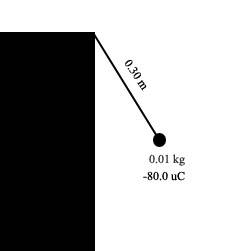M&MA wall has a negative charge distribution producing a uniform horizontal electric field. A small plastic ball of mass 0.01kg, carrying a charge of -80.0uC is suspended by an uncharged, nonconducting thread of 0.30m long. The thread is attached to the wall and the ball hangs in equilibrium, as shown above, in the electric and gravitational fields. The electric force on the ball has a magnitude of 0.032 N

a) Draw and label the forces acting on the ball
I already know how to do this

b) Calculate the magnitude of the electric fields at the ball's location due to the charge wall.
I used E= F(electric)/q and got -400 N/C as the answer

c) Determind the perpendicular distance from the wall to the center of the ball.
I'm stuck at this one. I knew it has something to do with breaking things into component then find the angle between the thread and the wall. If I know the angle I can use trigonometry to solve for the distance. But ahh! I don't know how to find the angle.

d) The string is now cut:
a) Calculate the magnitude of the resulting acceleration of the ball, and state its direction.
I have no idea what to do with this one

b) Describe the resulting path of the ball.
Well, for this one I think it will fall down eventually but curve to the right.

Please help me with these questionLast edited:

dicerandom
Good work so far, you're very close to the solution :)

In part (a) you should have three forces: the gravitational force, the electric force, and the force of tension from the string. The tensional force will have both x and y components, determined by the magnitude of the tension times the cosine and sine of the angle which the string makes with the horizontal.

If you use the fact that the ball isn't going anywhere and therefore the sum of the forces must be zero you should be able to solve for both the magnitude of the tension in the string and what the angle must be.

For part (d) the force of tension has been removed. What does this mean with regards to the sum of the forces acting on the ball?

M&M
Thanks a lot for the help. But I'm still stuck/confuse at some part hahaha...so is the tension times sine of the angle is the x component and tension (T) times the cosine of the angle is the y component?

Also in part d, I know there are 2 type of acceleration there. One in the x and one in the y direction. The y direction one is 9.8 since it's the gravity. But I still don't know how to find the one that act in the x direction. I was thinking about using F(net)=ma but what's the F(net)

sorry about all the questiondicerandom
For the x & y components of the tension, it depends on what angle you're using. From the equations for the components you gave that would be the angle which the string makes with respect to the vertical wall in your figure.

After the string is cut we've removed the force of tension, so the only two forces left are gravity and the electrostatic force.